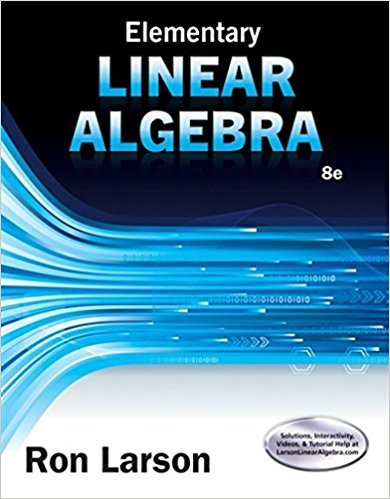×

×

Textbooks / Math / Elementary Linear Algebra 8

# Elementary Linear Algebra 8th Edition - Solutions by Chapter## Full solutions for Elementary Linear Algebra | 8th Edition

ISBN: 9781305658004Elementary Linear Algebra | 8th Edition - Solutions by Chapter

Solutions by Chapter
4 5 0 307 Reviews
##### ISBN: 9781305658004

Elementary Linear Algebra was written by and is associated to the ISBN: 9781305658004. This textbook survival guide was created for the textbook: Elementary Linear Algebra, edition: 8. Since problems from 45 chapters in Elementary Linear Algebra have been answered, more than 222080 students have viewed full step-by-step answer. This expansive textbook survival guide covers the following chapters: 45. The full step-by-step solution to problem in Elementary Linear Algebra were answered by , our top Math solution expert on 01/12/18, 03:19PM.

Key Math Terms and definitions covered in this textbook
• Adjacency matrix of a graph.

Square matrix with aij = 1 when there is an edge from node i to node j; otherwise aij = O. A = AT when edges go both ways (undirected). Adjacency matrix of a graph. Square matrix with aij = 1 when there is an edge from node i to node j; otherwise aij = O. A = AT when edges go both ways (undirected).

• Associative Law (AB)C = A(BC).

Parentheses can be removed to leave ABC.

• Augmented matrix [A b].

Ax = b is solvable when b is in the column space of A; then [A b] has the same rank as A. Elimination on [A b] keeps equations correct.

• Characteristic equation det(A - AI) = O.

The n roots are the eigenvalues of A.

• Complex conjugate

z = a - ib for any complex number z = a + ib. Then zz = Iz12.

• Determinant IAI = det(A).

Defined by det I = 1, sign reversal for row exchange, and linearity in each row. Then IAI = 0 when A is singular. Also IABI = IAIIBI and

• Eigenvalue A and eigenvector x.

Ax = AX with x#-O so det(A - AI) = o.

• Fast Fourier Transform (FFT).

A factorization of the Fourier matrix Fn into e = log2 n matrices Si times a permutation. Each Si needs only nl2 multiplications, so Fnx and Fn-1c can be computed with ne/2 multiplications. Revolutionary.

• Free variable Xi.

Column i has no pivot in elimination. We can give the n - r free variables any values, then Ax = b determines the r pivot variables (if solvable!).

• Gram-Schmidt orthogonalization A = QR.

Independent columns in A, orthonormal columns in Q. Each column q j of Q is a combination of the first j columns of A (and conversely, so R is upper triangular). Convention: diag(R) > o.

• Hilbert matrix hilb(n).

Entries HU = 1/(i + j -1) = Jd X i- 1 xj-1dx. Positive definite but extremely small Amin and large condition number: H is ill-conditioned.

• Nilpotent matrix N.

Some power of N is the zero matrix, N k = o. The only eigenvalue is A = 0 (repeated n times). Examples: triangular matrices with zero diagonal.

• Orthogonal matrix Q.

Square matrix with orthonormal columns, so QT = Q-l. Preserves length and angles, IIQxll = IIxll and (QX)T(Qy) = xTy. AlllAI = 1, with orthogonal eigenvectors. Examples: Rotation, reflection, permutation.

• Particular solution x p.

Any solution to Ax = b; often x p has free variables = o.

• Polar decomposition A = Q H.

Orthogonal Q times positive (semi)definite H.

• Pseudoinverse A+ (Moore-Penrose inverse).

The n by m matrix that "inverts" A from column space back to row space, with N(A+) = N(AT). A+ A and AA+ are the projection matrices onto the row space and column space. Rank(A +) = rank(A).

• Row picture of Ax = b.

Each equation gives a plane in Rn; the planes intersect at x.

• Simplex method for linear programming.

The minimum cost vector x * is found by moving from comer to lower cost comer along the edges of the feasible set (where the constraints Ax = b and x > 0 are satisfied). Minimum cost at a comer!

• Symmetric matrix A.

The transpose is AT = A, and aU = a ji. A-I is also symmetric.

• Toeplitz matrix.

Constant down each diagonal = time-invariant (shift-invariant) filter.